# PSAT Math : How to use scientific notation

## Example Questions

### Example Question #63 : Exponential Operations

Express 0.000000076 in scientific notation.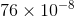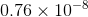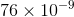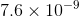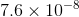Explanation:

Write the number: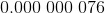Counting the number of places, move the decimal point to the right until it is after the first nonzero digit: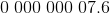The resulting number is 7.6; the number of places the decimal point moved to the right was 8. In scientific notation, this number is.

### Example Question #64 : Exponential Operations

Express the square of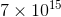in scientific notation.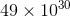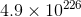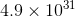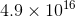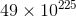Explanation:

Take advantage of the exponent rules.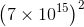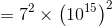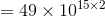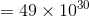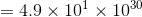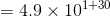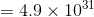### Example Question #65 : Exponential Operations

The number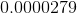is equivalent to which of the following?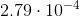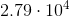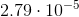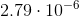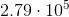Explanation:

Each of the answers to this question are in scientific notation. To determine the exponent on the 10, see how many places you need to move the decimal over to get from your original number to 2.79.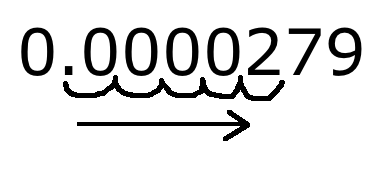Because the decimal place moves 5 places, the exponent will be 5, and because the decimal moves to the RIGHT, the exponent is going to be negative.

So the answer to the quesiton is### Example Question #66 : Exponential Operations

Put the following value in scientific notation: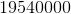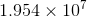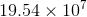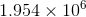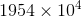Our first value for scientific notation should be betweenand. We then multiply this value byraised to a power equal to the number of spaces our decimal has moved to the left.